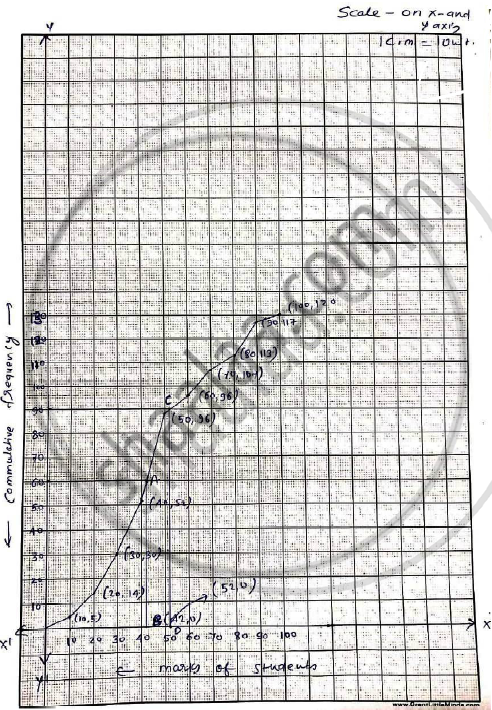Use Graph Paper for this Question. the Marks Obtained by 120 Students in an English Test Are Given Below : Draw the Ogive and Hence, Estimate: (I) the Median Marks. - Mathematics

Sum

Use graph paper for this question.
The marks obtained by 120 students in an English test are given below :

 Marks 0 - 10 10 - 20 20 -  30 30 - 40 40 - 50 50 - 60 60 - 70 70 - 80 80 - 90 90  -100 No.of students 5 9 16 22 26 18 11 6 4 3

Draw the ogive and hence, estimate:
(i) the median marks.
(ii) the number of students who did not pass the test if the pass percentage was 50.
(iii) the upper quartile marks.

SolutionC.I Marks lessthan No.ofstudents Cumulativefrequency 0-10 10 5 5 10-20 20 9 14 20-30 30 16 30 30-40 40 22 52 40-50 50 26 78 50-60 60 18 96 60-70 70 11 107 70-80 80 6 113 80-90 90 4 117 90-100 100 3 120

i. No.of students =120
∴ Median = 60th term through marks of 60 draw a line parallel to x - axis which meets the curve at A. From A draw perpendicular to x-axis, which meets it at A . From A draw a perpendicular to x-axis which
meets it at B.
ii. The upper quartile marks (Q_3 ) = 3/4 n^(th) term

= 3^(th)/4 xx 120= 90^(th)  term

draw a line parallel to x-axis which meets the 1 curve at C. From C draw perpendicular to x-axis which meets it at D.

Concept: Graphical Representation of Ogives
Is there an error in this question or solution?